Next: Cylindrical Airfoils Up: Incompressible Aerodynamics Previous: Introduction

# Theorem of Kutta and Zhukovskii

Consider a two-dimensional airfoil that is at rest in a uniform wind of speedwhose direction subtends a (clockwise) anglewith the negative-axis. It follows that the wind velocity is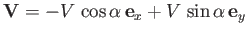, and the corresponding complex velocity is. (See Section 6.4.) The air velocity a great distance from the airfoil must tend toward this uniform velocity. Thus, for sufficiently large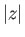, we can write (see Section 6.4)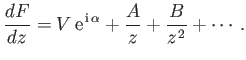(9.3)

According to Equation (6.172), the circulation,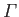, of air about the airfoil is determined by performing the integral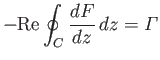(9.4)

around a loop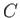that lies just above the airfoil surface. However, as discussed in Section 6.10, the value of this integral is unchanged if it is performed around any loop that can be continuously deformed onto, while not passing through the airfoil surface, or crossing a singularity of the complex velocity,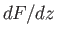(i.e., a line source or a-directed vortex filament). Because (in the high Reynolds number limit in which the boundary layer and the wake are infinitely thin) there are no line sources or-directed vortex filaments external to the airfoil, we can evaluate the integral around a large circle of radius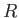, centered on the origin. It follows that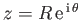and. Hence,(9.5)

which implies that(9.6)

at large.

As discussed in Section 6.11, the net force (per unit length) acting on the airfoil,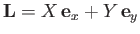, is determined by performing the Blasius integral,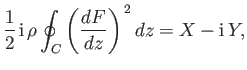(9.7)

around a loopthat lies just above the airfoil surface. However, as before, the value of the integral is unchanged if instead we perform it around a large circle of radius, centered on the origin. Far from the airfoil,(9.8)

So, we obtain(9.9)

or(9.10)

In other words, the resultant force (per unit length) acting on the airfoil is of magnitude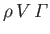, and has the direction obtained by rotating the wind vector through a right-angle in the sense opposite to that of the circulation. This type of force is known as lift, and is responsible for flight. The result (9.10) is known as the theorem of Kutta and Zhukovskii, after the German scientist M.W. Kutta (1867-1944), and the Russian scientist N.E. Zhukovskii (1847-1921), who discovered it independently. Note that (at fixed circulation) the lift is independent of the shape of the airfoil. Furthermore, according to the Kutta-Zhukovskii theorem, there is zero drag acting on the airfoil (i.e., zero force acting in the direction of the wind). In reality, there is always a small friction drag due to air viscosity, as well as a (hopefully) small form drag due to residual separation of the boundary layer from the back of the airfoil. There is actually a third type of drag, known as induced drag, that is discussed in Section 9.8.

As discussed in Section 6.11, the net moment per unit length (about the origin),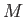, acting on the airfoil is determined by performing the integral(9.11)

around a loopthat lies just above the airfoil surface. As before, we can deforminto a circle of radius, centered on the origin, without changing the value of the integral. Hence, we obtain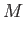(9.12)

or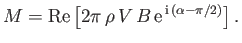(9.13)Next: Cylindrical Airfoils Up: Incompressible Aerodynamics Previous: Introduction
Richard Fitzpatrick 2016-03-31